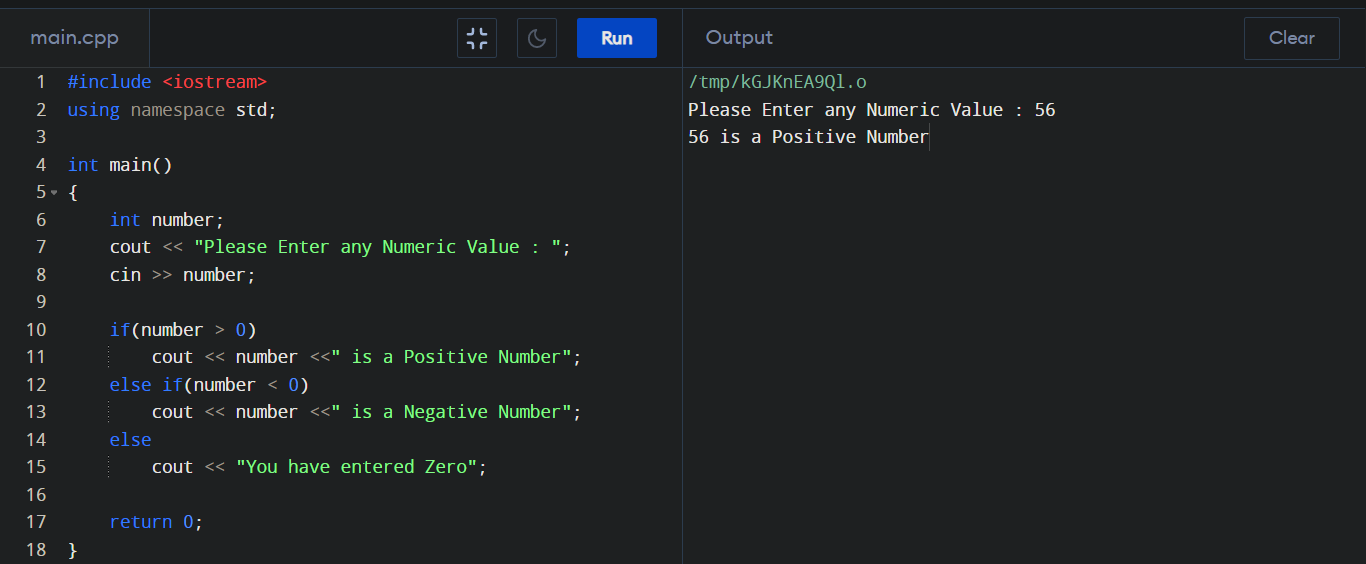C++ Program to check Number (Positive or Negative or Zero)

How to check if a Number is Positive or Negative or Zero? By this following program we can do this. Here we used "if-else" statements to check the Number type.

Positive or Negative checking by C++ :

#include <iostream>
using namespace std;

int main()
{
int number;
cout << "Please Enter any Numeric Value : ";
cin >> number;

if(number > 0)
cout << number <<" is a Positive Number";
else if(number < 0)
cout << number <<" is a Negative Number";
else
cout << "You have entered Zero";

return 0;
}

Output :